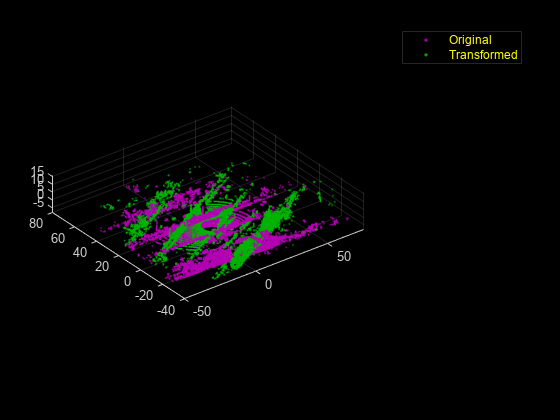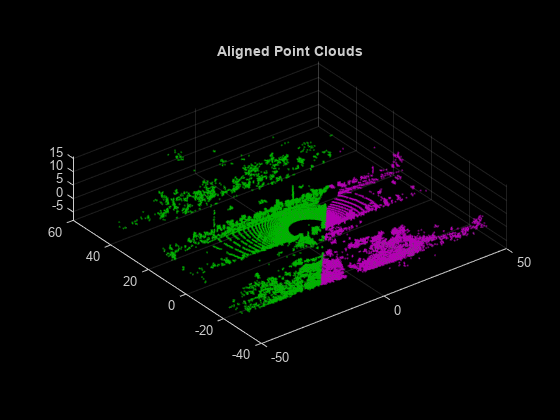# Estimate Transformation Between Two Point Clouds Using Features

This example shows how to estimate a rigid transformation between two point clouds. In the example, you use feature extraction and matching to significantly reduce the number of points required for estimation. After you use the `extractFPFHFeatures` function to extract fast point feature histogram (FPFH) features from the point clouds, you use the `pcmatchfeatures` function to search for matches in the extracted features. Finally, you use the `estimateGeometricTransform3D` function and the matching features to estimate the rigid transformation.

### Preprocessing

Create two point clouds by applying rigid transformation to an input point cloud.

Read the point cloud data into the workspace.

```rng("default") ptCld = pcread("highwayScene.pcd"); ptCld.Count```
```ans = 65536 ```

Downsample the point cloud to improve the computation speed, as it contains around 65,000 points.

```ptCloud = pcdownsample(ptCld,"gridAverage",0.2); ptCloud.Count```
```ans = 24596 ```

Create a rigid transformation matrix with a 30-degree rotation and translation of 5 units in x- and y-axes.

```rotAngle = 30; rot = [cosd(rotAngle) sind(rotAngle) 0; ... -sind(rotAngle) cosd(rotAngle) 0; ... 0 0 1]; trans = [5 5 0]; tform = rigid3d(rot,trans);```

Transform the input point cloud.

`ptCloudTformed = pctransform(ptCloud,tform);`

Visualize the two point clouds.

```pcshowpair(ptCloud,ptCloudTformed) xlim([-50 75]) ylim([-40 80]) legend("Original","Transformed","TextColor",[1 1 0])```### Feature Extraction and Registration

Extract features from both the point clouds using the `extractFPFHFeatures` function.

```fixedFeature = extractFPFHFeatures(ptCloud); movingFeature = extractFPFHFeatures(ptCloudTformed);```

Find matching features and display the number of matching pairs.

```[matchingPairs,scores] = pcmatchfeatures(fixedFeature,movingFeature, ... ptCloud,ptCloudTformed,"Method","Exhaustive"); length(matchingPairs)```
```ans = 1814 ```

Select matching points from the point clouds.

```fixedPts = select(ptCloud,matchingPairs(:,1)); matchingPts = select(ptCloudTformed,matchingPairs(:,2));```

Estimate the transformation matrix using the matching points.

```estimatedTform = estimateGeometricTransform3D(fixedPts.Location, ... matchingPts.Location,"rigid"); disp(estimatedTform.T)```
``` 0.8660 0.5000 0.0002 0 -0.5000 0.8660 -0.0002 0 -0.0003 0.0000 1.0000 0 4.9995 5.0022 0.0020 1.0000 ```

Display the defined transformation matrix.

`disp(tform.T)`
``` 0.8660 0.5000 0 0 -0.5000 0.8660 0 0 0 0 1.0000 0 5.0000 5.0000 0 1.0000 ```

Use the estimated transformation to retransform `ptCloudTformed` back to the initial point cloud.

`ptCloudTformed = pctransform(ptCloudTformed,invert(estimatedTform));`

Visualize the two point clouds.

```pcshowpair(ptCloud,ptCloudTformed) xlim([-50 50]) ylim([-40 60]) title("Aligned Point Clouds")```## SupportGet trial now